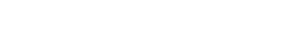Drag

# 统计测试代写｜STAT2006 Assignment 3

## Question 1.

Observations {𝑋1,𝑋2, … , 𝑋100} be an independent random sample from 𝑛𝑛(𝜃, 0.09), where 𝜃 is an unknown parameter. Consider the following hypothesis testing

𝐻0: 𝜃 = 1.2 versus 𝐻1: 𝜃 ≠ 1.2.

Suppose the rejection region is given by |X− 1.2| > 0.0588.

(a)Compute the level of significance.

(b)What is the probability of type II error in terms of 𝜃𝜃?

(c)Suppose that we observe X= 1.26, calculate the p-value.

## Question 2.

A car has an average fuel consumption of 33 mpg (with 𝜎𝜎 = 5.7). We took the new model of the car out for 35 tests and collected the data giving 𝑥=34.8 mpg. Assume the new model’s fuel consumption is normally distributed and has the same 𝜎𝜎, we want to decide whether this new model has better fuel consumption than the original one. (mpg stands for miles per gallon).

(a)Given the null hypothesis and alternative hypothesis based on the above description.

(b)Choose an appropriate test statistic.

(c)Give the critical (rejection) region and p-value analysis respectively based on the data collected under 5% level of significance.

## Question 3.

There are two identical coins, each with a probability 𝑝 ∈ (0,1) to turn up a head when tossed.To test if the coins are fair, an experiment was performed 20 times. For the 𝑖𝑡ℎ experiment,the coins were tossed together, and denote 𝑋𝑖 = 1 if they turned up differently (one head and one tail), and 𝑋𝑖 = 0 otherwise.

(a)Specify the null and alternative hypothesis in terms of the unknown parameter 𝑝.

(b)Taking X as the test statistic, find a critical (rejection) region for the specified hypothesis test, with significance level 5%.

(c)When 𝑝 = 0.75, find the probability of the Type II error of the test specified in part(b).

## Question 4.

Suppose the ages of customers of a discount store chain follows a normal distribution is generally behaved to have variance 𝜎𝜎2 = 50. Given a random sample of 8 customers with the following ages 38, 46, 30, 35, 29, 40, 40, 46. Do critical (rejection) region analysis and p-value analysis under 5% level of significance for the following hypothesis of testing

𝐻0: 𝜎2 = 50 vs 𝐻1: 𝜎2 < 50.

## Question 5.

Assume that Peet’s Coffee is looking at two possible locations for a new shop. To help make a decision they look at the number of pedestrians that go by each of the two locations in one hour segments. The results of the random sample are shown in the following table. Assume the two population variances are not known but equal. Complete the steps required to test the null and alternative hypothesis at the 1% level of significance that both sites have the same number of pedestrians and calculate the 95% confidence interval.Question 6.

Consider a random sample {𝑋𝑋1, 𝑋𝑋2, … , 𝑋𝑋𝑛𝑛}drawn from an exponential distribution with rate𝜆>0.

(a)Show that 2𝑛λ𝜒~𝜒22𝑛.

(b)Using 𝜒 as the test statistic, find a decision rule for testing 𝐻0: 𝜆 = 𝜆0 vs 𝐻1: 𝜆 > 𝜆0 at 𝛼 significance level.

(c)Suppose that 𝜆0 = 1. Find the p-value for the test found in part (a), given the sample {1.38, 0.02, 0.04,0.48, 0.75}.